## Su Doku

Su Doku is usually played on a 9 by 9 board, divided into 3 by 3 cells. You can generalize this into playing on an M×M square board broken into non-overlapping rectangular cells, each containing M squares (an obvious question is to how define a puzzle where M is a prime number). The solution of the puzzle is to place M symbols on the board such that each row, column or cell contains each symbol exactly once, without moving the initial clues. Puzzles with M<9 are called Sub Dokus, those for M>9 are called Super Dokus.

Technorati tag:

Related topic: Kakuro

Thanks to the following people who pointed out errors and generally helped me keep this page of tips in tip-top shape: Larry (LA, CA, USA), Justin Pearson, Tom Schoenemann (Michigan, USA), Mike Fleming (New Ipswich, New Hampshire, USA), Mike Godfrey (Manchester, UK), G B (whose name I wish I knew).

In this page: Guides, Tips and tricks, Methods and madness.

# How to solve Su Doku: tips

## The mathematics of Su Doku

### Guides

1. Roger Walker's tips were the first set I ever found. They take you from nothing into formal reasoning methods with colourful names like "three in a bed" at your own pace. What I liked best about this site are the animated tutorials.
2. Michael Mepham's article from the Daily Telegraph is by now somewhat of a classic. It is a very well-written systematic explanation of the basic techniques for solving Su Doku. It introduced the nomenclature "Ariadne's thread".
3. Angus Johnson's Simple Sudoku web site has a very fine page of Su Doku tips, starting with the most basic element: find the singletons, and progressing to complicated and bizarrely named rules of Su Doku logic like the "Swordfish".
4. Dan Rice's Sudoku Blog also does a good job of explaining the rules of inference, one per blog. Since it is a blog, it takes each rule by itself and spends time explaining it. It contains some of the best explanations I have seen of the more complex rules.
5. SuDokuHints has a nice tool: it can give hints on a puzzle, solve one step while telling you how it was done, or solve the full puzzle and explain each step. You can play games that it generates, or type your own puzzle into it.

### My own two bits: a fiendish tutorialWhy another tutorial? Because you don't really need to know many tricks. I show this using a relatively hard puzzle by Wayne Gould, who creates puzzles for "The Times" of London. These are rated in difficulty from mild (the simplest) to fiendish (the one on the left). Gould claims that none of his puzzles ever need trial and error solutions. If you follow this example through you will find that you never really need very complicated tricks either. Another way of solving this very puzzle is given by Roger Walker in one of his tutorials. Our methods differ: I try to illustrate some often-used tricks in this example.

#### Step 1: Singletons: find the "loner"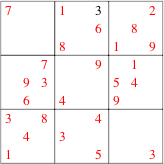"When you have eliminated the impossible whatever remains, however improbable, is the truth", said Sherlock Holmes. This is the principle by which we put the 3 in the top row. 1, 2 and 7 are eliminated by the clues in the row; 4, 5, 6 and 9 by those in the column, and 8 by the cell. This leaves the truth. I don't see it as very improbable; but one must give the master some poetic license. This rule may or may not be useful to begin things off, but it is indispensible in the end game (especially when it is coupled with the hidden loner rule of Step 8).

#### Step 2: Basic "slice and dice"Let's see how to place a 4 in the bottom right cell. The blue lines show that it must go right into the bottom-most row, because the other two rows already have a 4 in them. These are the slices. Now one of the three squares in the bottom row of the cell already has a clue in it. The other square is eliminated by dicing. The green line shows that the middle column is ruled out, because it already contains a 4 in another cell. So we have finished the second move in a fiendish puzzle and found out what slicing and dicing is.

#### Step 3: Applied "slice and dice"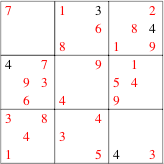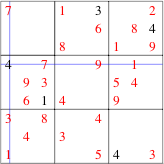We can place two more 4s, shown in black in the picture on the left. This requires slice and dice exactly as before. Another example: we can place a 1 by slice and dice as shown in the picture on the right.

#### Step 4: Simple "hidden pairs"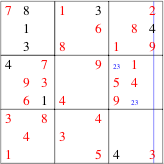Angus Johnson has this to say about hidden pairs: "If two squares in a group contain an identical pair of candidates and no other squares in that group contain those two candidates, then other candidates in those two squares can be excluded safely." In the example on the right, a 2 and a 3 cannot appear in the last column. So, in the middle rightmost cell these two numbers can only appear in the two positions where they are "pencilled in" in small blue font. Since these two numbers have to be in these two squares, no other numbers can appear there.

#### Step 5: "Locked candidates"

Angus Johnson again: "Sometimes a candidate within a cell is restricted to one row or column. Since one of these squares must contain that specific candidate, the candidate can safely be excluded from the remaining squares in that row or column outside of the cell." Since the hidden pair 2 and 3 prevent anything else from apearing in the first two columns of the middle rightmost cell, an 8 can only appear in the last column. Now we apply the locked candidates rule.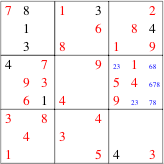We want to place an 8 in the bottom right cell. The last column can be sliced out by the locked candidate rule. Other slicing and dicing is normal, leading to the placement of the 8 as shown.

#### Step 6: Bootstrap by extending the logic of "locked candidates"

To get to the first step of the bootstrap from the last picture shown above, we need to slice and dice to place an 8 in the center bottom cell. You must be an expert at this method by now, so I leave that in your capable hands.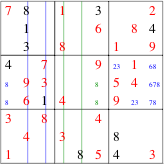The first element of the bootstrap is to place 8s in the middle row of cells. The picture on the left shows where the 8s must be placed in the middle left cell. The picture on the right shows the placement in the central cell.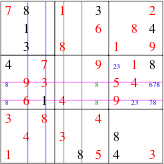Next we extend the logic of the locked candidates. The 5th and 6th rows must each have an 8: one of them has it in the middle left cell, and the other in the central cell. Therefore the 8 in the middle right cell cannot be in either of these rows. From what we knew before, the 8 must be in the top right corner square of the cell, as shown in the picture on the right. This is almost magical. Putting together imprecise information in three different cells, we have reached precise information in one of the cells.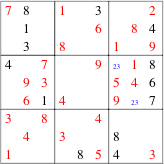And now the final step of the bootstrap is shown in the picture on the left. The placement of the 8 dictates that the 6 must be just below it, and therefore the 7 in the remaining square. The diabolical magic is complete: reason enough for this to be classified as a fiendish puzzle. One of Roger Walker's tutorials is a solution of precisely this puzzle, by a different route. But before going there, I invite you to try your hand at completing the solution which we have started upon here.

#### Step 7: The beginning of the end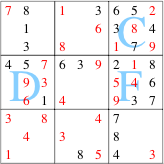The worst is over. We are now truly into the end game. First complete cell C entirely by the "loner" trick: filling 6, 5, 3 and 7 in that order. Next complete the cell F. Then finish the 7th column, place the 5 in the cell D, and complete that row, in order to get the picture on the left. We are more than half done. From now on common sense prevails: fill things in one by one. Don't panic, there are no sharks circling the boat. No swordfish either.

#### Step 8: "Hidden loner": almost not worth naming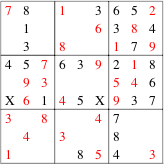The last rule, I promise. And it is hardly one, although you could call it the "hidden loner" rule. The only reason one should give it a name is that it fixes this very useful method in one's mind. So here is the example: In the 6th row there's more than one choice in each square. However there is only one place where the 5 can go (it is excluded from the squares with X's in them). So there is a loner hidden in this row: hence the name. I stop here, but you can go on to solve a fiendish puzzle by the simplest tricks exclusively.

### Not so fiendish?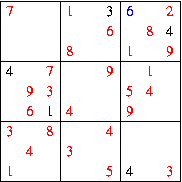Mike Godfrey wrote to me to point out a much simpler way of solving this particular puzzle. After step 3, as before, one can fill in the 6 shown in blue in the figure here, by noting that all other numbers can be eliminated by requiring that they do not appear in the same row, column or block. After this the remaining puzzle can be solved by spotting singles.

Mike writes that this puzzle "is not too fiendish perhaps". Perhaps. But that opens up the question of how to rate puzzles. I haven't found much discussion of this aspect of the mathematics of Su Doku: partly because commercial Su Doku generators (by that I mean the humans behind the programs) are not exactly forthcoming about their methods, but also because the problem is not terribly well-defined. This is a wide open field of investigation.

### From tricks to methods: the roots of mathematics

#### Constraint programmingThe minimum Su Doku shown alongside (only 17 clues) requires only two tricks to solve: identifying hidden loners and simple instances of locked candidates. The key is to apply them over and over again: to each cell, row and column. The application of constraints repeatedly in order to reduce the space of possibilities is called constraint programming in computer science. "Pencilling in" all possible values allowed in a square, and then keeping the pencil marks updated is part of constraint programming. This point has been made by many people, and explored systematically by Helmut Simonis.

#### Non-polynomial state space

This is where much of the counting appears. Before clues are entered into a M×M Su Doku puzzle, and the constraints are applied, there are MM2 states of the grid. This is larger than any fixed power of M (this is said to be faster than any polynomial in M). If depth-first enumeration were the only way of counting the number of possible Su Dokus, then this would imply that counting Su Doku is a hard problem. Application of constraints without clues is the counting problem of Su Doku. As clues are put in, and the constraints applied, the number of possible states reduces. The minimum problem is to find the minimum number of clues which reduces the allowed states to one. The maximum problem is analogous.

Many known hard problems are of a type called nondeterministic-polynomial. In this class, called NP, generating a solution of a problem of size M takes longer than any fixed power of M, but given a solution, it takes only time of order some fixed power of M to check it (ie, a polynomial in M). If enumeration were the only way of counting the number of Su Doku solutions, then this would be harder than NP. If someone tells me that the number of Su Doku solutions is 6670903752021072936960, I have no way to check this other than by counting, which I know to takes time larger than polynomial in M. At present there is no indication that the counting problem of Su Doku is as easy as NP.

#### Trial-and-error: is Su Doku an NP complete problem?

The Su Doku problem is to check whether there is an unique solution to a given puzzle: the yes/no answer would usually, but not necessarily, produce the filling of the grid which we call a solution. It would be in NP if the time an algorithm takes to solve the M×M Su Doku problem grows faster than any fixed power of M. It is not known whether the Su Doku problem is in NP.

One sure fire way of solving any Su Doku puzzle is to forget all these tricks and just blindly do a trial-and-error search, called a depth-first search in computer science. When programmed, even pretty sloppily, this can give a solution in a couple of seconds. If we use this method on M×M Super Doku, then the expected run time of this program on the trickiest puzzles (called worst-case in computer science) would grow faster than any fixed power of M, but (of course) it is guaranteed to solve the puzzle. If trial-and-error were the only algorithm to solve any Su Doku puzzle whatsoever, and one were able to show that the state space of a puzzle grows faster than a fixed power of M, then this would prove that Su Doku is an NP problem.

Helmut Simonis has results which might indicate that trial-and-error is never needed, and a small bag of tricks with hyper arc consistency always answers the Su Doku question. However, one needs to ask how many times the consistency check has to be applied to solve the worst-case problem, and how fast this grows with M, in order to decide whether constraint programming simplifies the solution.

#### The controversy over trial-and-error

From this formal point of view, one can see the debate raging currently on Michael Mepham's web site and other discussion boards on Su Doku as an argument between the search enthusiasts and the constraint programming wallahs: with Mepham slowly giving ground in his defence of search. But does the debate just boil down to choosing which algorithm to use? Yes, if the Su Doku problem is easy (ie, in P) and constraint programming solves it faster. However, if Su Doku is hard, then there is a little more to it.

#### Backdoors: defining "satisfactory puzzles"

In many instances of NP complete problems, the average run time of programs can be substantially less than the worst-case. Gomes and Selman conjecture that this is due to the existence of "backdoors", ie, small sets of tricks which solve these average problems. Here human intuition (called heuristics in computer science) can help to identify the backdoors and often crack the nut faster than the sledge hammer of systematic algorithms. These I call "satisfactory puzzles". One of the open problems for Su Doku is to define precisely the nature of such backdoors, and the classes of problems which contain them.

#### Zen and the art of gardening

We have introduced elsewhere a method of counting Su Dokus by a depth first enumeration of trees (called the garden of forking paths). It is clear that some of the branches of these trees are much longer than the average. As M grows, this imbalance also grows (polynomially, or faster?). This is one way of visualizing the difference between the average case (satisfactory puzzles) and the worst case (diabolical puzzles). My challenge is a gardening problem: how do you make the trees come out balanced and symmetric? It is like a Zen puzzle: if you solve it, then you reduce human intuition (heuristics) to an algorithm; even if it is impossible you gain insight by contemplating the problem.

© Sourendu Gupta. Mail me if you want to reproduce any part of this page. My mailing address is a simple (satisfactory) puzzle for you to solve. Created on 13 October, 2005. Last modified on 19 June, 2006.